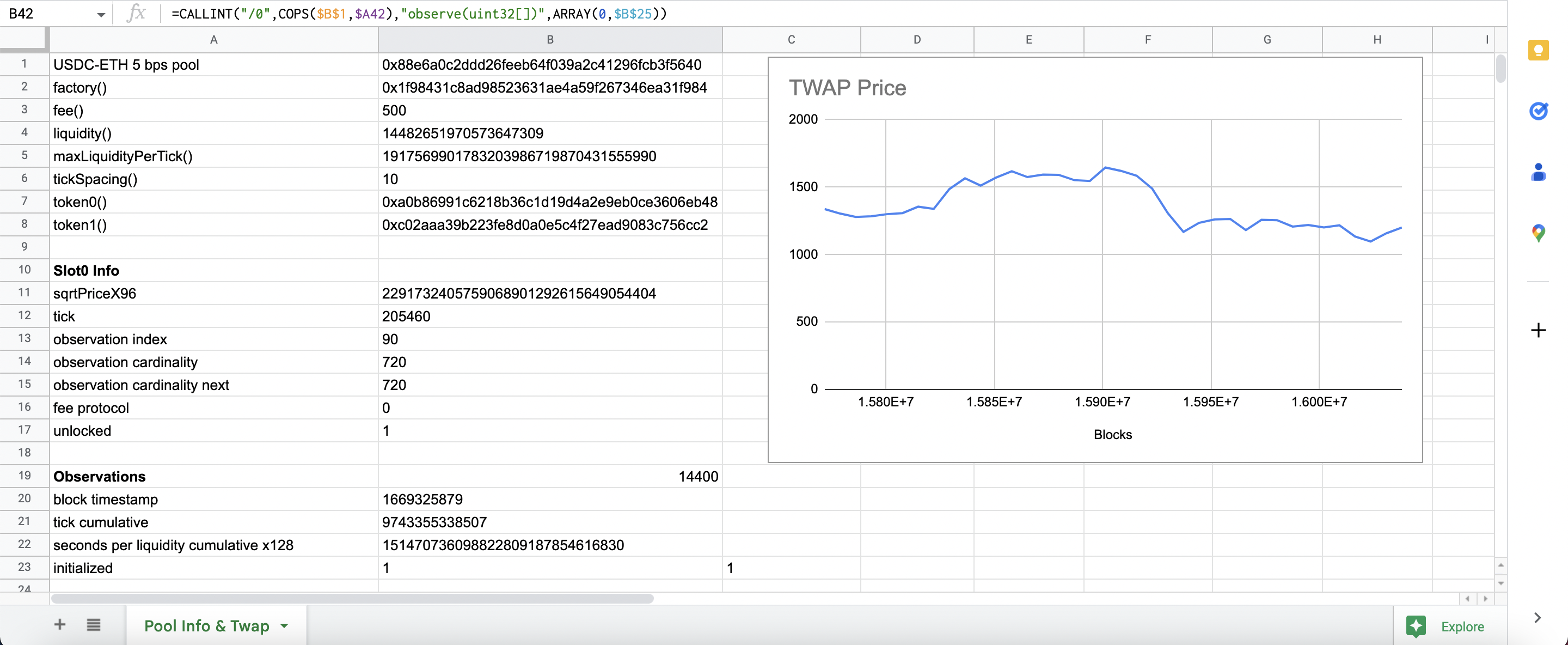# Uniswap v3

Web3 Sheets Demo: Uniswap### Example Explanation​

This demonstration displays the time weighted average price of WETH within the Uniswap v3 pool featuring functions that call the liquidity, protocol fee, and other aspects of the ool listed below.

Note: the function names are in the A column, which is why each of the below functions references an A cell.

• B2 `=CALLADDRESS("",B1,A2)` - returns the factory address of the pool.

• B3 `=CALLINT("",B1,A3)` - returns the fee amount for the pool.

• B4 `=CALLINT("",B1,A4)` - returns the liquidity for the pool.

• B5 `=CALLINT("",B1,A5)` - returns the max liquidity per tick for the pool.

• B6 `=CALLINT("",B1,A6)` - returns the tick spacing for the pool.

• B7 `=CALLADDRESS("",B1,A7)` - returns the token0 address for the pool.

• B8 `=CALLADDRESS("",B1,A8)` - returns the token1 address for the pool.

Slot 0 Info

• B11 `=CALLINT("0",B1,"slot0()")` - returns the sqrtPriceX96 for the pool.

• B12 `=CALLINT("1",B1,"slot0()")` - returns the tick for the pool.

• B13 `=CALLINT("2",B1,"slot0()")` - returns the observation index.

• B14 `=CALLINT("3",B1,"slot0()")` - returns the observation cardinality.

• B15 `=CALLINT("4",B1,"slot0()")` - return the observation cardinality next.

• B16 `=CALLINT("5",B1,"slot0()")` - returns the protocol fee.

Observations

• B20 `=CALLINT("0",B1,"observations(uint256)",\$B\$13)` - returns the last observation timestamp.

• B21 `=CALLINT("1",B1,"observations(uint256)",\$B\$13)` - returns the tick cumulative.

• B22 `=CALLINT("2",B1,"observations(uint256)",\$B\$13)` - returns the seconds per liquidity cumulative x128.

• B23 `=CALLINT("3",B1,"observations(uint256)",\$B\$13)` - returns where the tick has been initialized.

TWAP

• D26 and below `=((sqrt(1.0001^((C26-B26)/\$B\$25))*(2^96)*1000000000)/(2^96))^2*10^-6` - returns a 24 minute TWAP price of the pool and utilzes the COPS handler to make historical TWAP calls.